# Vertical land movements

Home Products (demonstrative) Vertical land movements
GPS
ALTIMETRY
•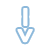• ULR NGL JPL GFZ

## GNSS solutions

### Solution : ULR7A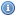• Legend
• (mm/year)
•> +6
•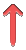> +4
•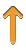> +2
•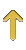> +1
•> +0.5
•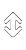-0.5/+0.5
•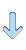< -0.5
•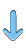< -1
•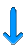< -2
•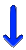< -4
•< -6
•GPS@TG
•RF & GPS@TG
•RF
•OTHER

The ULR7a GPS solution is a preliminary version of the reanalysis of 21 years of GPS data from 2000 to 2020 that has been undertaken within the framework of the 3rd data reprocessing campaign of the International GNSS Service (IGS). Its associated vertical velocity field is expressed in ITRF2014.

Double-differenced ionosphere-free GPS carrier phase observations from daily regional (plus one global) networks of 546 stations were reanalyzed using through a free-network strategy (station positions, Earth Orientation parameters, satellite orbits and zenith tropospheric delays adjusted simultaneously using GAMIT software. The daily subnetworks were then combined into daily global network of stations using GLOBK software. The data analysis strategy (models, corrections...) was compliant with the specifications adopted by the IGS for this reanalysis (more information here).

Position time series expressed in ITRF2014 were then computed using CATREF software using a time-dependent functional model that includes station positions at reference epoch, velocities, semi-annual and annual seasonal signals and transformation parameters (translation, rotation, scale, and their velocities) between the daily undetermined frames and the ITRF2014 for a subset of IGS core stations. Where appropriate, station position offsets (mostly due to equipment changes or earthquakes), velocity changes and post-seismic displacement signals were added.

After the substraction of non-tidal atmospheric loading displacements in the time series (provided by the Earth System Modelling team of the German research center for geosciences at Potsdam), both a functional and a stochastic model were adjusted including long-term linear trends, position offset discontinuities, and periodic signals, following the equation:

\begin{align} x(t) = & x_{ref}+ v_{x}(t-t_{ref}) & \textit{reference position and velocity}\\ & + \sum_{i=1}^{N_{O}} a_{i}H(t-t_{i}) & \textit{position offsets}\\ & + \sum_{j=1}^3 s_{j}\sin(\frac{2\pi}{\tau_{j}}t)) + c_{j}\cos(\frac{2\pi}{\tau_{j}}t)) & \textit{seasonnal signals} \\ & + \sum_{d=1}^8 s_{d}\sin(\frac{2\pi}{\tau_{d}}t)) + c_{d}\cos(\frac{2\pi}{\tau_{d}}t)) & \textit{draconitic signals}\\ & + \sum_{f=1}^3 s_{f}\sin(\frac{2\pi}{\tau_{f}}t)) + c_{f}\cos(\frac{2\pi}{\tau_{f}}t)) & \textit{fortnightly signals} \\ & + \sum_{k=1}^{N_{PSD}}PSD_{k}(t) & \textit{post-seismic deformation signals} \end{align}

where:

\begin{align} x_{ref} & \text{ is the position at the reference epoch} t_{ref} \\ v_{x} & \text{ is the linear velocity} \\ H(t-t_{i}) = & \begin{cases} 0 & \text{if } t \lt t_{i}\\ \\ \frac{1}{2} & \text{ if } t = t_{i}\\ \\ 1 & \text{if } t \gt t_{i} \end{cases} \\ \tau_{j} = & \frac{1}{j} \text{ years} \\ \tau_{d} = & \frac{P_{D}}{365.25} \text{ years}, P_{D} \text{ being the period in days of the draconitics} \\ \tau_{f} = & \frac{P_{F}}{365.25} \text{ years}, P_{F} \text{ being the period in days of the fortnightly signals}\\ PSD_{k}(t) = & \begin{cases} a_{k} \log(1+ \frac{t-t_{k}}{\tau_{k}}) \text{ if PSD model is log} \\ \\ a_{k}(1- \exp(-\frac{t-t_{k}}{\tau_{k}})) \text{ if PSD model is exp} \\ \\ a_{1k} \log(1+ \frac{t-t_{k}}{\tau_{1k}}) + a_{2k}(1- \exp(-\frac{t-t_{k}}{\tau_{2k}})) \text{ if PSD model is log+exp} \\ \\ a_{1k} \log(1+ \frac{t-t_{k}}{\tau_{1k}}) + a_{2k} \log(1+ \frac{t-t_{k}}{\tau_{2k}}) \text{ if PSD model is log+log} \\ \\ a_{1k}(1- \exp(-\frac{t-t_{k}}{\tau_{1k}})) + a_{2k}(1- \exp(-\frac{t-t_{k}}{\tau_{2k}})) \text{ if PSD model is exp+exp} \end{cases} \end{align}

### Vertical velocity field

 Estimated (robust) velocities: 546 CGPS@TG 461 Average of formal errors: 0.38 mm/yr Median of formal errors: 0.28 mm/yrVertical velocities table

The ULR7a_Vertical-Velocities_Table provides the vertical GPS velocities and uncertainties for the 546 stations fulfilling the criteria of 3 years of minimum length without discontinuities and with data gaps not exceeding 30%.

The velocities come from the adjustment of both a functionnal and stochastic model, the latter accounting for a linear combination of white noise and power law process, whose parameters were estimated using the Restricted Maximum Likelihood Estimation method.Daily time series

The ULR7A_neu.zip file contains individual station data files of daily position time series in ITRF2014 with respect to the position at the reference epoch. These positions are expressed in meters in the local frame (North, East, and Up). The reference position and the 3D velocity in the local coordinate system (East, North, Up) are provided in the header of each file. .

The ULR7A_neu_model.zip file contains individual station data files of daily position time series predictions (i.e. modelled) in ITRF2014 with respect to the position at the reference epoch. These predictions are expressed in meters in the local frame (North, East, and Up). The reference position and the 3D velocity in the local coordinate system (East, North, Up) are provided in the header of each file. More information about the model used can be found in the About tab.Position discontinuities

The ULR7a_discontinuities_Table.txt file provides the position offsets that were estimated.

The ULR6b GPS solution is an updated version of ULR6a. That is, ULR6b is aligned in the ITRF2014 reference frame, instead of ITRF2008 for ULR6a. Both UMR6 solutions result from the reanalysis of 19 years of GPS data from 1995 to 2014, carried out within the framework of the 2nd reanalysis (reprocessing) campaign (repro2) of the International GNSS Service (IGS).

Double-differenced ionosphere-free GPS carrier phase observations from a global network of 756 stations were reanalyzed using GAMIT/GLOBK software version 10.5. The data set covered the period January 1995 to December 2013. Station coordinates, satellite orbits, Earth orientation parameters (EOPs), and zenith tropospheric delay parameters every hour, were estimated. The data analysis strategy (models, corrections...) was compliant with the specifications adopted by the IGS for this reanalysis (more information here). A detailed description of ULR data analysis strategy can be found in the reference below.

The GPS velocities were obtained in the final step of the analysis, which combined the daily GAMIT/GLOBK global network solutions (station coordinates with full co-variances) from the entire data time span into a long-term solution using CATREF software. This first long-term solution (ULR6a) was aligned to ITRF2008 (latest version of the ITRF available at that time) using minimal constraints over a selected set of IGS Reference Frame stations. Later on, this solution was aligned to the ITRF2014 using the official transformation parameters between ITRF2008 and ITRF2014.

### General

 Analysis center: ULR Number of stations: 674 Solution: ULR6B CGPS@TG 482 Date of publication: 2020 Reference Frame 194 Time span: 1995.0 - 2013.9 Others 77 Reference Frame: ITRF 2014

### Vertical velocity field

 Estimated (robust) velocities: 493 CGPS@TG 349 Average of formal errors: 0.54 mm/yr Median of formal errors: 0.36 mm/yr

To assign more realistic uncertainties on the GPS velocities, the noise content in the position time series was examined by the maximum likelihood estimation (MLE) technique using CATS software (Williams, 2008). Time series were detrended at the CATREF stage. Details on the GPS velocity uncertainty assessment are given in the reference below.Vertical velocities table

The ULR6b_Vertical-Velocities_Table provides the vertical GPS velocities and uncertainties for the 493 stations fulfilling the criteria of 3 years of minimum length without discontinuities and with data gaps not exceeding 30%.Daily time series

The ULR6B_neu.zip file contains individual station data files of daily position time series in ITRF2014 with respect to the position at the reference epoch. These positions are expressed in meters in the local frame (North, East, and Up). The reference position and the 3D velocity in the local coordinate system (East, North, Up) are provided in the header of each file.Position discontinuities

The ULR6b_discontinuities_Table.txt file provides the position offsets that were estimated.

### Citation

#### Please cite this paper if you find the results useful:

Gravelle, M., K. Gobron, G. Wöppelmann, et al. (in prep.). The ULR-repro3 GPS data reanalysis and its estimates of vertical land motion at tide gauges for sea level science. In preparation.

## GNSS solutions

### Solution : NGL14

• Legend
• (mm/year)
•> +6
•> +4
•> +2
•> +1
•> +0.5
•-0.5/+0.5
•< -0.5
•< -1
•< -2
•< -4
•< -6

The GNSS solution named ’NGL14’ is produced by the Nevada Geodetic Laboratory (NGL) using a Precise Point Positioning (PPP) data analysis strategy. The GipsyX software (version 1.0) from the Jet Propulsion Laboratory (JPL) is employed to produce daily position time series for over 17,000 globally distributed stations using JPL satellite orbits and clock products expressed in the IGS14 reference frame (Blewitt et al. 2018). The data set covers the period January 1996 to March 2022.

This GNSS data analysis strategy complies with the standards (models, corrections,...) of the International GNSS Service (IGS) reanalysis (reprocessing) campaigns ; hence the Sixteenth session of the Group of Experts for the Global Sea Level Observing System (GLOSS), held in Busan (Republic of Korea), 11-13 April 2019, tasked the SONEL data assembly centre to consider including the NGL solution (position time series and velocities) for the subset of GNSS stations nearby tide gauges. Among the more than 17,000 stations processed by NGL, as many as 1111 have actually been identified as co-located, or nearby, tide gauges.

The GNSS velocities, provided here for 1069 stations (nearby tide gauges and reference frame stations included) with a minimum record length of 3 years and data gaps not exceeding 30%, are produced by NGL using the MIDAS estimator (Blewitt et al. 2016).

More details about the GNSS data analysis strategy can be found here or in Blewitt et al. (2016).

### Vertical velocity field

 Estimated (robust) velocities: 1069 CGPS@TG 872 Average of formal errors: 0.83 mm/yr Median of formal errors: 0.67 mm/yrVertical velocities table

The NGL14_Vertical-Velocities_Table provides the vertical GPS velocities and uncertainties from the 1069 stations fulfilling the criteria of 3 years of minimum length and data gaps not exceeding 30%, estimated by the NGL group using MIDAS (Blewitt et al. 2016).Daily time series

The NGL14.zip file contains individual station data files of daily position time series in ITRF2014 with respect to the position at the mid time series epoch. These positions are expressed in meters in the local frame (North, East, and Up). The reference position and the 3D velocity (obtained using MIDAS) in the local coordinate system (East, North, Up) are provided in the header of each file.Position discontinuities

The NGL14_discontinuities_Table.txt file provides the position offsets that can affect the station position and velocity estimates, as indicated by the NGL group. Note that MIDAS should be robust regarding the velocity estimates in presence of offsets, but not the parametric methods (e.g. least squares adjustment).

More information and data sets useful for other applications can be found at the Nevada Geodetic Laboratory website.

### Citation

#### Please cite this paper if you find the results useful:

For the time series:
Blewitt G., W. C. Hammond, C. Kreemer (2018). Harnessing the GPS data explosion for interdisciplinary science. Eos, 99, doi:10.1029/2018EO104623.

For the velocities:
Blewitt G., C. Kreemer, W.C. Hammond, J. Gazeaux (2016). MIDAS robust trend estimator for accurate GPS station velocities without step detection. Journal of Geophysical Research, 121, 2054-2068, doi:10.1002/2015JB012552.

## GNSS solutions

### Solution : JPL14

• Legend
• (mm/year)
•> +6
•> +4
•> +2
•> +1
•> +0.5
•-0.5/+0.5
•< -0.5
•< -1
•< -2
•< -4
•< -6

The GNSS solution named ’JPL14’ is produced by the Jet Propulsion Laboratory (JPL) group using a Precise Point Positioning (PPP) data analysis strategy. JPL’s GipsyX software is employed to produce daily position time series for over 2,000 globally distributed stations using JPL satellite orbits and clock products expressed in the IGS14 reference frame. The data set covers the period January 1994 to April 2022.

This GNSS data analysis strategy complies with the standards (models, corrections,...) of the International GNSS Service (IGS) reanalysis (reprocessing) campaigns ; hence the Sixteenth session of the Group of Experts for the Global Sea Level Observing System (GLOSS), held in Busan (Republic of Korea), 11-13 April 2019, tasked the SONEL data assembly centre to consider including the JPL solution (position time series and velocities) for the subset of GNSS stations nearby tide gauges. Among the more than 2,000 stations processed by JPL, as many as 332 have actually been identified as co-located, or nearby, tide gauges.

The GNSS velocities, provided here for 436 stations (nearby tide gauges and reference frame stations included) with a minimum record length of 3 years and data gaps not exceeding 30%, are also produced by JPL.

More details about the GNSS data analysis strategy can be found here.

### Vertical velocity field

 Estimated (robust) velocities: 436 CGPS@TG 281 Average of formal errors: 0.42 mm/yr Median of formal errors: 0.32 mm/yrVertical velocities table

The JPL14_Vertical-Velocities_Table provides the vertical GPS velocities and uncertainties from the 436 stations fulfilling the criteria of 3 years of minimum length and data gaps not exceeding 30%, estimated by the JPL group.Daily time series

The JPL14.zip file contains individual station data files of daily position time series in ITRF2014 with respect to the position at the epoch 2023-01-01. These positions are expressed in meters in the local frame (North, East, and Up). The reference position and the 3D velocity in the local coordinate system (East, North, Up) are provided in the header of each file.Position discontinuities

The JPL14_discontinuities_Table.txt file provides the position offsets that have been estimated by the JPL group.

More information and data sets useful for other applications can be found at the JPL website.

### Citation

#### Please cite this paper if you find the results useful:

Heflin, M., Donnellan, A., Parker, J., Lyzenga, G., Moore, A., Ludwig, L. G., et al. (2020). Automated estimation and tools to extract positions, velocities, breaks, and seasonal terms from daily GNSS measurements: illuminating nonlinear Salton Trough deformation. Earth and Space Science, 7, e2019EA000644. https://doi.org/10.1029/2019EA000644

## GNSS solutions

### Solution : GT2

• Legend
• (mm/year)
•> +6
•> +4
•> +2
•> +1
•> +0.5
•-0.5/+0.5
•< -0.5
•< -1
•< -2
•< -4
•< -6

The GNSS solution named ’GT2’ is produced by the German Research Centre for Geosciences (GFZ) for the International GNSS Service (IGS) working group TIGA. It contains position time series for 757 permanent GNSS stations expressed in the ITRF2014 reference frame over the period January 1994 to December 2015.

This GNSS solution was carried out within the framework of the 2nd IGS reanalysis (reprocessing) campaign (Repro2). Among the 757 stations processed by GFZ, as many as 538 have actually been identified as co-located, or nearby, tide gauges.

The GNSS velocities, provided here for 579 stations (nearby tide gauges and reference frame stations included) with a minimum record length of 3 years and data gaps not exceeding 30%, are also produced by GFZ. To be more realistic, constant values (0.1 mm/yr in horizontal and 0.3 mm/yr in vertical) have been added to the formal errors on the velocities.

More details about the GNSS data analysis strategy can be found in Deng et al. (2015).Vertical velocities table

The GT2_Vertical-Velocities_Table provides the vertical GPS velocities and uncertainties from the 593 stations fulfilling the criteria of 3 years of minimum length and data gaps not exceeding 30%, estimated by the GFZ group.Daily time series

The GT2_neu.zip file contains individual station data files of daily position time series in ITRF2014 with respect to the position at the mid time series epoch. These positions are expressed in meters in the local frame (North, East, and Up). The reference position and the 3D velocity in the local coordinate system (East, North, Up) are provided in the header of each file.Position discontinuities

The GT2_discontinuities_Table.txt file provides the position offsets that have been estimated by the GFZ group.

### Citation

#### Please cite this paper if you find the results useful:

Deng Z., Gendt G., Schöne T. (2015) Status of the IGS-TIGA Tide Gauge Data Reprocessing at GFZ. In: Rizos C., Willis P. (eds) IAG 150 Years. International Association of Geodesy Symposia, vol 143. Springer, Cham https://link.springer.com/chapter/10.1007%2F1345_2015_156

•• AVISO CCI CSIRO

## Analyses of Sea Level Differences (Satellite altimetry minus tide gauge data)

• Legend
• (mm/year)
•> +6
•> +4
•> +2
•> +1
•> +0.5
•-0.5/+0.5
•< -0.5
•< -1
•< -2
•< -4
•< -6Observational situation near to the coast

In briefest terms, the approach consists in differencing the sea level time series from a tide gauge with an equivalent time series from satellite altimetry. To the extent that both instruments measure identical ocean signals, their difference is a proxy for the vertical position of the tide gauge. Assuming that the instrumental drifts are negligible, the time series of the sea level differences will then be dominated by vertical land motion at the tide gauge. This is illustrated in the figure on the right (click on the image to enlarge).

It is essential that the processing of both data types be as consistent as possible too. For instance, since an atmospheric correction is typically applied to satellite altimetry data, we applied the identical correction to the tide-gauge data. This correction is referred to as SLP (Sea Level Pressure) correction in the header of the t-files, but it comprises the static and dynamic effects of the atmosphere. The corrections were provided by AVISO under the name Dynamic Atmospheric Correction (DAC). However, the details of the atmospheric corrections and their accuracies are not relevant here, since common errors will cancel in the differentiation. It is the consistency of models and corrections applied to both datasets that must be ensured.Tide gauge data:
All tide gauge data used in our calculations are from the Revised Local Reference (or ’RLR’) dataset of the PSMSL. We selected tide gauges with more than 15 years and a minimum of 70% of valid monthly mean sea levels over the satellite altimetry period, starting in 1993.0.Satellite altimetry data:
We used time series of satellite altimetry mean sea level anomalies (SLA), i.e., sea surface heights with respect to an arbitrary temporal mean (it can be different from one provider to the other), whose primary raison d’être is to avoid large numerical values (decametric ellipsoidal heights with regard to the expected centimeter level changes in mean sea levels). Note that we considered satellite altimetry data from several data suppliers.

Here, we are dealing with the data set identified as Global MSLA heights in delayed time ("all sat merged") from the Archiving, Validation, and Interpretation of Satellite Oceanographic data (AVISO; http://www.aviso.altimetry.fr/en/data.html). The temporal sampling was homogenized to time series of monthly data.Sea level differences:
Time series of sea level differences (SLD) between monthly tide gauge and satellite altimetry data were built using three variants of satellite SLA time series, namely the closest grid point, the most correlated grid point, and the average within a 1° radius from the tide gauge location. However, since the differences between these variants were negligible, we adopted the SLA averaged within a radius of 1° around the tide gauge location.

Once computed, the time series of sea level differences (SLD) were checked visually to discard those that displayed clear non linear behaviour, i.e., for which vertical land motion would not be adequately modelled by a linear trend. We then estimated the linear trend of the de-seasoned and de-trended SLD time series. All quoted uncertainties represent 1-sigma standard errors.Future updates:
We plan to update the calculations of the sea level differences on a yearly basis, because as the time series lengthen and the satellite providers improve their products (new models, corrections, algorithms...), some level of improved precision is expected. However, there can be real differences between sea levels measured by a tide gauge at the coast and that measured off-shore by a satellite altimeter, due to the ocean processes which occur between the two points. This could be interesting to investigate and monitor. By contrast, for most open ocean locations, there is usually a high degree of correlation between variability in the two types of sea level. In this case, the vertical land motion can be estimated, provided the instrumental drifts are negligible. If there are (drifts), it enables tide gauge data to be used as a check on the satellite altimeter information and its stability and, conversely, it enables gross errors in tide gauge datum to be identified.

The web map enables the users to navigate from one site (station) to another, and click on a specific site in the map to see its attributes. By clicking further, an individual web page for the site can be accessed, where details on the data and meta-data are displayed for that site. The user can then get some additional statistics and download files with the associated time series (sea level differences...). Here, we provide a more comprehensive means to download the data from all the sites.Table of linear trends of sea level differences

The vertical_velocities_ALTG_AVISO.txt provides the vertical velocities and uncertainties for the 478 stations for which we computed the monthly sea level differences (satellite altimetry minus tide gauge data) and derived a linear trend (see About).Time series of monthly sea level differences

The useries_aviso.zip archive (.zip file) contains the 478 individual files of monthly sea level differences (satellite altimetry minus tide gauge data) time series. The format of the individual files is rather straightforward. The values are expressed in millimetres with respect to an arbitrary datum. The choice of the datum was arbitrary to avoid negative values (a constant of about 7000 mm was added to the differences).

### Citation

#### Please cite this paper if you find the results useful:

G. Wöppelmannn & M. Marcos (2016) : Vertical land motion as a key to understanding sea level change and variability. Reviews of Geophysics, 54, pp. 64-92.

## Analyses of Sea Level Differences (Satellite altimetry minus tide gauge data)

Work in progress...

## Analyses of Sea Level Differences (Satellite altimetry minus tide gauge data)

Work in progress...